# Image processing

Brain Simulator contains several image processing nodes and algorithms. So far, it includes GPU implementation of the SLIC algorithm , basic algorithm for joining super-pixels, image patch selection algorithm, and on-line k-means clustering that constitute a working memory in our example.

The image processing nodes will be presented on the example of ball-tracking in the Breakout game.

## The method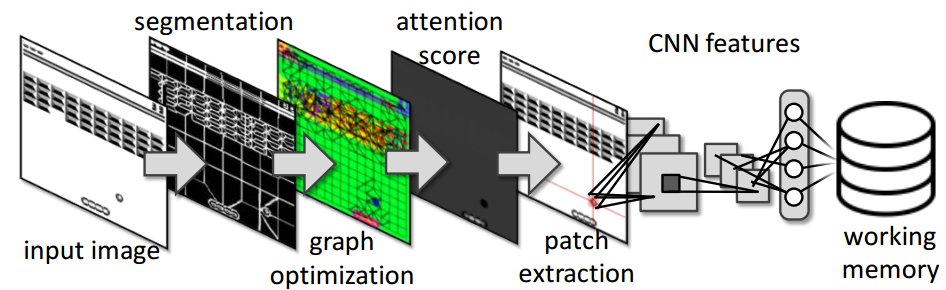The algorithm drives its attention from one object to another and it works in the following way. First, an input image is segmented into super-pixels (SP) using the SLIC  method. Second, each SP is connected with its neighbors and close-by SP are assigned into a same object id. Third, the attention energy ($E_a$) is estimated for each object. Fourth, features are estimated for the object with the highest $E_a$ by a hierarchy of convolutions and fully-connected layers. Fifth, the object features are clustered into a Visual Words  to constitute a Working Memory.

### Super-pixels pre-processing

SLIC algorithm [1,2] was used to divide image into a set of super-pixels (SP). Important is that the algorithm is fast, each SP covers roughly uniform area. It also assigns a simple descriptor to each super pixel based on its average color and its change in time. An Example of usage of the segmentation node is shown in the image below. It requires to set up the Params/nSegs parameter (right side of the figure) that defines the number of segments. Number of pixels in the image has to be divisible by the number segments (nSegs). So far, the input image has to be always square.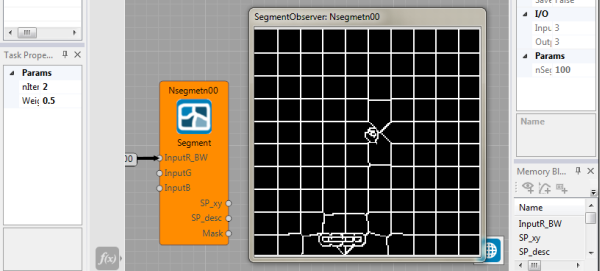### Join patches

Super-pixels are now concatenated into more-meaingful sets, similar to objects. The goal is to find which neighborhood super-pixels correspond to the same object and which do not. Thus, a graph of super-pixel connections is created. Each edge (that connects vicinity super-pixels) is weighted based on the difference between the super-pixel descriptors. If the weight of the edge is larger than Params/Threshold, super-pixels that are connected by the edge belongs to the same object.

Result of this node has same format as its input, but indexes of segments are now different as they correspond to the object proposals.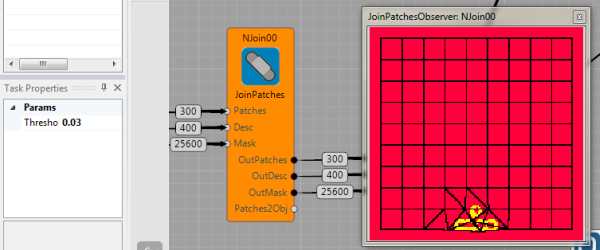The algorithm now decides on which object it focuses its attention. Thus, each object has an attention value $E_{a}(o_i)$ that concatenates the fact that we want to focus on stuff that moves, but we want to focus on different objects too. It is an energy-like function calculated for each object in this way: $E_{a}(o_i) = E_{time}(o_i) + \alpha E_{move}(o_i)$, where $E_{time}$ decreases in time and increases only if it was just chosen, $E_{move}$ is from the change in time descriptor.

### Representing the object

In the example file, the image patch is represented only by the raw pixels, richer version that is based on the pre-learn features is in the Breakout game scheme. In the richer version, the focuser output (image patch) is used as an input into a following neural network and the back-propagation algorithm is used to learn the network (Auto-encoder in this case) to represent the data . In this example, the network is created from two convolutional layers and one fully-connected layer. The last layer is a vector representation of the object.

In the simple image processing example, the object is represented as a raw image patch. It is visualized in the figure below. The Focuser node is used to visualized the the location and size of the selected object (observer on the left side in the figure bellow). Its output is raw image (middle observer i the figure below), then we used the resize node to get the desired resolution (right observer).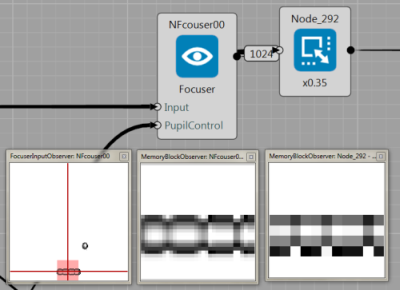### Visual memory of objects

MyKMeansWM node clusters all objects, the method is following. For the given focuser output, we compare it with the current state of the memory and if it is similar to an object, we update the object based on the given input data. If not, it creates the new object. The method can be seen as an on-line version of the popular K-Means clustering algorithm [5,6].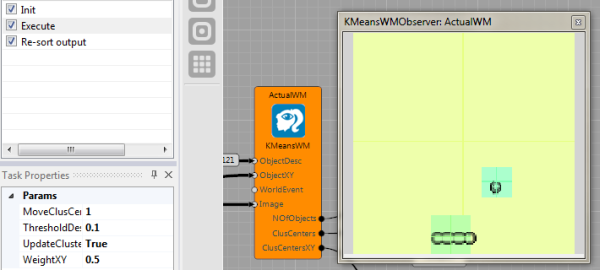Overall, the final scheme for the vision part is implemented in Brain Simulator as: